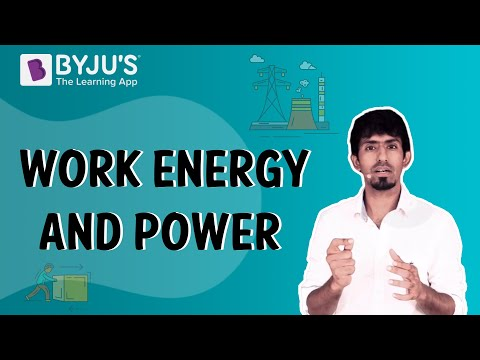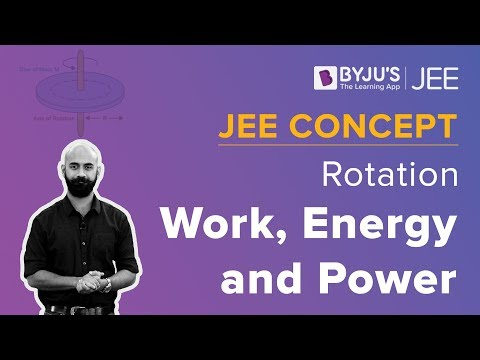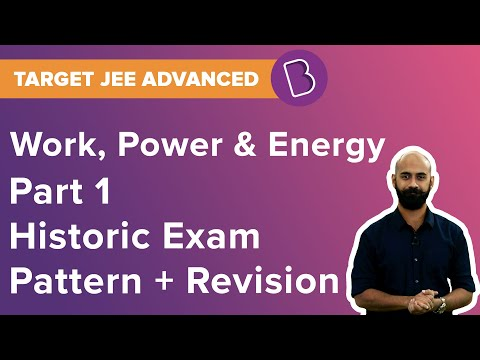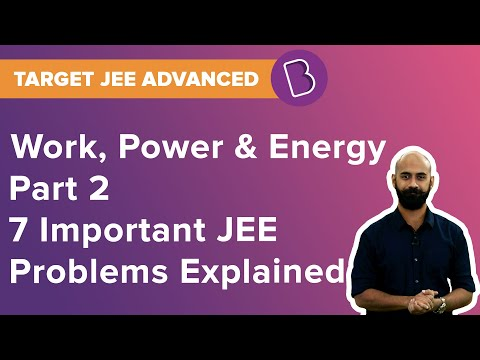# Work-Energy And Power Class 11 Notes

 List of Content What is Work? What is Power? What is Energy? What is the Principle of Conservation of Energy? Important Questions

## CBSE Class 11 Physics Work-Energy And Power Notes

Work, power, and energy are among the most important concepts of mechanics. A moving car, walking up stairs, lifting heavy objects, an aeroplane flying, etc are among the most common examples that give us insight into the concept of work, power, and energy. There are few common notions of work, power, and energy that can be precisely defined in physics. These notions and measurements can be utilized to define and predict the motion and behavior of bodies.

### What is Work?

Work is the measurement of energy transfer that takes place when an object is displaced by an external force applied in the direction of the displacement.

### What is Power?

The rate of doing work is called power.

### What is Energy?

The capacity for doing work is called Energy. It may exist in kinetic, potential, thermal, chemical, electrical, nuclear, or various other forms. The SI unit of energy is the same as that of work.

#### For More Information On Work, Energy and Power, Watch The Below Videos:### What is the Principle of Conservation of Energy?

The Principle related to the conservation of mechanical energy states that the total mechanical energy of a substance remains in equilibrium if the only forces acting upon the body are conservative in nature.

#### Law Of Conservation Of Energy

Energy can neither be created nor be destroyed however, it can be changed from one to another form. The total energy in an isolated system remains the same or energy is conserved over time. The mass remains the same in any closed system within boundaries except for the heat form which can cross boundaries.

As per the law, energy cannot be created and neither can be destructed but conveniently can be changed to different forms. Chemical energy can be converted into heat energy which could further be used to generate potential energy in a different body. As energy is changed from one to another form, net gain/loss remains the same. Therefore, energy is said to be conserved wherein the total amount remains the same.

### Work Energy And Power Important Questions

1. The sign of work done by a force on a body is important to understand. State carefully if the following quantities are positive or negative:
• (a) work done by a man in lifting a bucket out of a well by means of a rope tied to the bucket.
• (b) work done by gravitational force in the above case,
• (c) work done by friction on a body sliding down an inclined plane,
• (d) work done by an applied force on a body moving on a rough horizontal plane with uniform velocity,
• (e) work done by the resistive force of air on a vibrating pendulum in bringing it to rest.
1. Underline the correct alternative :
• (a) When a conservative force does positive work on a body, the potential energy of the body increases/decreases/remains unaltered.
• (b) Work done by a body against friction always results in a loss of its kinetic/potential energy.
• (c) The rate of change of total momentum of a many-particle system is proportional to the external force/sum of the internal forces on the system.
• (d) In an inelastic collision of two bodies, the quantities which do not change after the collision is the total kinetic energy/total linear momentum/total energy of the system of two bodies.

#### Work Power and Energy Questions Explained, Watch The Below Videos:These work energy and power class 11 notes pdf can be viewed below:

The students can prepare all the important concepts included in this unit by referring to the work energy and power class 11 notes pdf from the links mentioned below:

• Work Energy and Power Class 11 notes pdf
• Energy
• Work
• Types of Energy
• Heat Energy and Internal Energy
• Different Forms Of Energy
• Power
• Conventional and Non-conventional Energy Sources
• Kinetic Energy
• Electric Power
• Potential energy

Access CBSE class 11 Physics Sample here.

Access NCERT Books for class 11 Physics here.

## Frequently asked Questions on CBSE Class 11 Physics Notes: Work Energy Power

### What is the ‘Law of conservation of energy’?

The law of conservation of energy states that the amount of energy is neither created nor destroyed.

### What is ‘Work’?

The measure of energy transfer that occurs when an object is moved over a distance by an external force is known as ‘Work’.

### What is the definition of ‘Power’?

Power can be defined as the rate of doing work.